Transition metals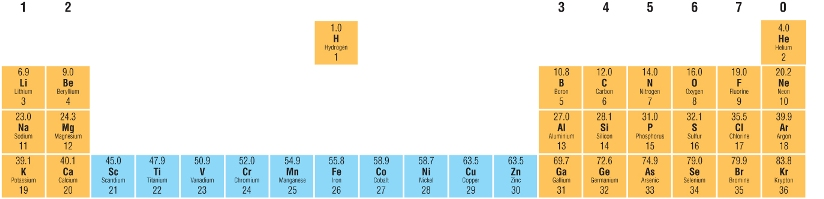# A Transition element is a d - block element that forms at least one ion with an incomplete d - sub shell.

• This means that Scandium and Zinc are not Transition elements.

• It also explains why compounds of these are white and not coloured.

Sc        1s22s22p63s23p63d14s2                       Sc3+        1s22s22p63s23p6

Zn        1s22s22p63s23p63d104s2                      Zn2+        1s22s22p63s23p63d10

Writing electron configurations

• Remember this is the arrangement of electrons in their orbitals.

• Remember the 4s  - sub shell fills before the 3d - sub shell: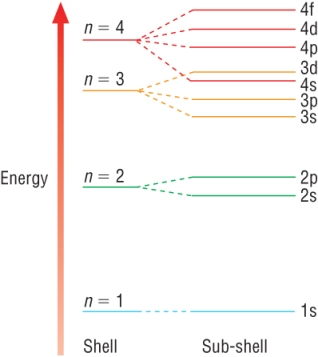• The electrons fill in order remember but their are 2 anomalous elements.

• Chromium and Copper fills differently:Cr:      1s22s22p63s23p63d44s2  (expected)      Cr:      1s22s22p63s23p63d54s1  (actual) Cu:      1s22s22p63s23p63d94s2  (expected) Cu:      1s22s22p63s23p63d104s1  (actual)
• This shows that these atoms show anomalous stability

A half - filled or full d - sub shell offers more stability than a full s - sub shell.

The electron configurations of ions:

• As metals, the Transition elements lose electrons forming ions when they react.

• When forming ions:

4s - sub shell empties before 3d - sub shell

• This seems a bit odd as the 4s fills first.

• When the orbitals have been filled the 4s and 3d sub shell levels swap over in the expected order.

• This happens because the energy levels are very close in the first place.

• The addition of electrons changes the energy levels slightly so they swap over (line emission spectra).

• It is easier to do if you write the electron configuration in electron shell order:

Example 1

Fe:          1s22s22p63s23p63d64s2

Fe3+:      1s22s22p63s23p63d5

Example 2

Cu:         1s22s22p63s23p63d104s1

Cu2+:     1s22s22p63s23p63d9

Qu 1-2  P203

Physical properties - general metals

• Shiny

• High densities

• High melting points and boiling points

• Giant metallic structure

• Delocalised electrons - good conductors of electricity

Physical properties - transition metals

• Variable oxidation states

• Coloured solutions

• Catalysts - due to d shell electrons

Variable oxidation states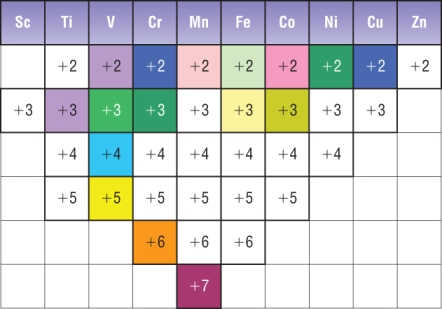Transition elements can exist in multiple oxidation numbers. The most common is 2+ as the 4s electrons are usually the first to go. Because the 4s and 3d electrons are close in energy, the 3d electrons can be easily removed as well This means they can form several ions by losing different numbers of electrons, all of which are fairly stable.  Changes in oxidation states of the transition metals often give rise to colour changes during the reaction.

MAKE SURE YOU CAN WORK OUT OXIDATION STATES

Recap:  Rules for assigning Oxidation Numbers

1)    Ox. No. of an element = 0

2)    Ox. No. of each atom in a compound counts separately.  Sum = 0

3)    Ox. No. of an ionic element = charge on ion.

4)    In a polyatomic ion (SO42-), The sum of the Ox. No.’s of the atoms = charge on ion.

5)    H = +1  except with metals (metal hydrides = -1).

6)    Gp 7 (Halogens) = -1  (except with oxygen)

7)    O = -2  except in peroxides  (H2O2,  O = -1)

Examples:

 1) KMnO4: K Mn O x 4 = 0 +1 ? 8- = 0 +7
 2) K2Cr2O7: K x 2 Cr x 2 O x 7 = 0 +2 2 x ? 14- = 0 +6

Coloured compounds:

• Transition metal solutions are coloured because they absorb wavelengths of white light from the visible spectrum.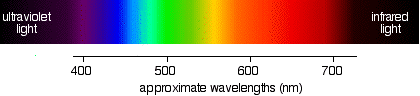• All other wavelengths are able to travel through the solution.
• Simply put: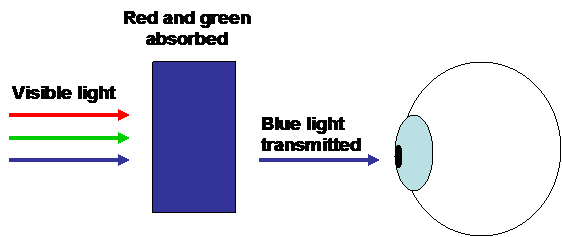• The colours are linked to the incomplete d shell.
•                         Sc3+:         1s22s22p63s23p6

No partially filled d shell = no colour.  Sc3+ is white

Qu 1 - 3  P205

Catalysis and precipitation

Transition metals as catalysts

• A catalyst speeds up a reaction and comes out unchanged, by providing an alternative route with a lower activation energy.
• Transition metals and their compounds are good catalysts
• There are 2 ways they act as catalysts:

1)    Transferring electrons easily:

• Because transition metals have many oxidation states, they can provide then accept electrons easily.
• Acting a bit like a middle man they can provide electrons at the start, then accept them at the end (or vice versa)
• In this way, they can bind to reactants forming part of the intermediate.
• This provides an alternative route offering a lower activation energy.

2)    Providing a site to react:

• Transition metals have the ability to bond to a wide range of molecules and ions.
• This means a reacting molecule can be held in place by adsorption while the reaction occurs.
• When the reaction is complete, the products desorb.
• It acts as a 'dating agency' providing 'introductions'.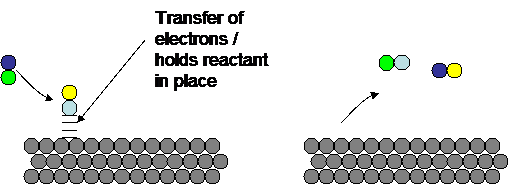Haber process:

 N2(g) + 3H2(g) D 2NH3(g)
• Fe catalysts increases the rate allowing a lower temperature to be used.  Used to make fertilisers.

Contact process:

 2SO2(g) + O2(g) D 2SO3(g)
• V2O5 catalyst increases the rate.  Used to make Sulphuric acid which is used to make detergents, explosives etc

Hydrogenation of alkenes: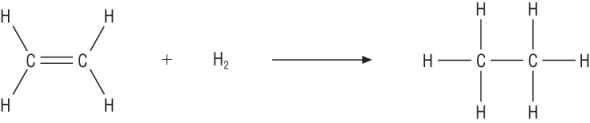• Nickel catalyst increases the rate meaning a lower temperature and pressure can be used.  Used in the hydrogenation of oils.

Decomposition of hydrogen peroxide:

 2H2O2(aq) à 2H2O(l) + O2(g)
• This reaction occurs very slowly at room temperature.
• MnO2 catalyst increases the rate.  Used to make oxygen gas.

Precipitating reactions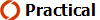• Precipitation reactions are reactions where soluble reactants form an insoluble product.
• The hydroxides of transition metal ions are a gelatinous precipitate which are insoluble and coloured.
• The addition of dilute sodium hydroxide is often used to identify the transition metal:
 Reaction Notes Solution colour Precipitate colour Cu2+(aq) Pale blue + 2OH-(aq) à Cu(OH)2(s) Blue ppt Co2+(aq) Pink + 2OH-(aq) à Co(OH)2(s) Blue ppt u Turns beige in the presence of air Fe2+(aq) Green + 2OH-(aq) à Fe(OH)2(s) Green ppt u Turns brown at surface in the presence of air [O] Fe3+(aq) Yellow + 3OH-(aq) à Fe(OH)3(s) Rusty - brown ppt

Qu 1 - 4  P207

Transition metals and complex ions

Complex ions

• A property of transition metals is their ability to form complex ions: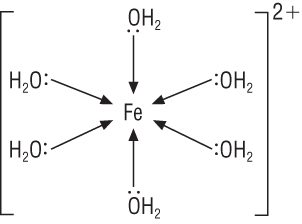Complex ion: Central metal ion is surrounded by ligands Ligand: Molecule / ion which donates a pair of electrons forming a coordinate bond (dative bond) Coordinate bond: One of the bonded atoms has provided both electrons for the covalent bond

In the example above:

• Fe 2+ is the metal ion.

• Ligands are the water molecules.

• Coordination number is the number of coordinate bonds to the central metal ion = 6.

• Square brackets groups the species and the overall charge is written outside the brackets.

• Overall charge is the sum of the charges of the metal ion and the ligands (if the ligands have a charge)

Common ligands:

 Ligand Formula Charge Water :OH2 0 Ammonia :NH3 0 Thiocyanate :SCN- -1 Cyanide :CN- -1 Chloride :Cl- -1 Hydroxide :OH- -1
• Ligands have a pair of electrons that  donors which is used to make a dative covalent bond to the central metal ion.

• All the ligands in the table have 1 lone pair and can form 1 dative covalent bond with the central metal ion.

• These types of ligands are called monodentate.

• The number of dative bonds a ligand is able to form is reflected in the prefix – mono, bi, hexa (poly) etc.

Examples:

1)        [Ti(H2O)6]3+

 No Ligands  x  charge + No Ligands  x  charge = Total charge of ligands + Total charge on ion = Total charge on complex ion 5  x  0 + - = 0 + ? = 3+

The central metal ion must be:    Ti3+

2)       [Co(H2O)5Cl]1+

 No Ligands  x  charge + No Ligands  x  charge = Total charge of ligands + Total charge on ion = Total charge on complex ion 5  x  0 + 1  x  1- = 1- + ? = 2+

The central metal ion must be:    Co2+

Shapes of complex ions:

• Complex ions have different shapes.
• The most common is octahedral formed by 6 ligands (coordination number of 6)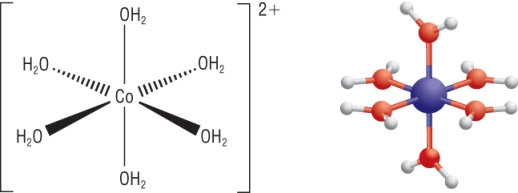Qu 1 - 3

Stereoisomerism in complex ions

What is stereoisomerism?

They have the same structural formula but with a different spatial arrangement

• This has been covered before in AS with:

Cis / Trans isomerism

Optical isomerism

Cis / Trans isomerism

• These occur in:

1)    Octahedral with 4 of one ligand and 2 of another ligand, [Co(NH3)4Cl2]+: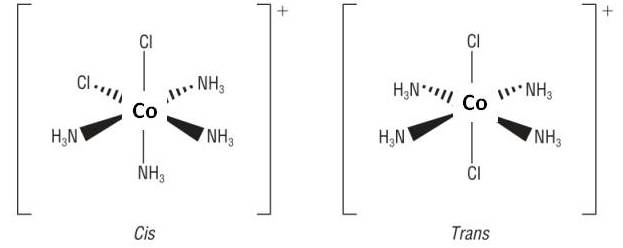CIS = 2 ligands are at 90o to each other TRANS = 2 ligands are at 180o to each other

2)    Square planar with 2 of one ligand and 2 of another ligand: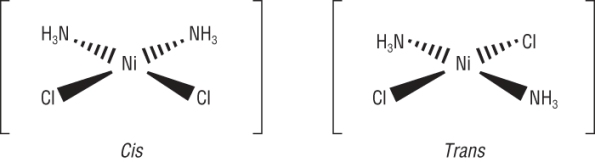CIS = 2 ligands are at 90o to each other TRANS = 2 ligands are at 180o to each other

Transition metal complexes in medicine: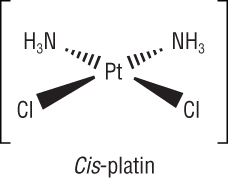Cis platin, [PtCl2(NH3)2], is the most effective drug against cancer. It binds to the DNA of fast - growing cancer cells preventing them from replicating. The cells own repair system leads to the death of the cells. This is the basis for chemotherapy, it has some very unpleasant side effects.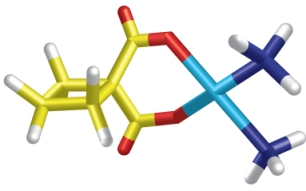New treatments involve carboplatin.  This has fewer side effects and lower doses can be used.

Qu 1 - 2  P211

Bidentate and multidentate ligands

Bidentate ligands

• Some ligands contain 2 lone pairs of electrons forming 2 coordinate bonds each.

• Ethane - 1,2 -  diamine,   H2NCH2CH2NH2     abbreviated to 'en':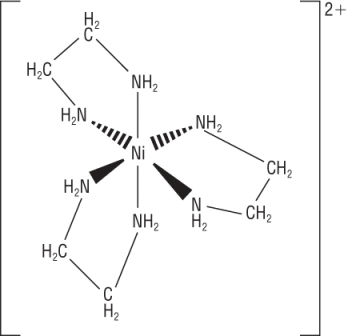[Ni(en)3]2+ Coordination number = 6 Octahedral

Cis - trans isomerism from bidentate ligands:

• Bidentate ligands exhibit cis trans isomerism with an octahedral shape.

• Ethanedioate ligand: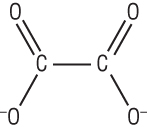• [Cr(C2O4)2(H2O)2]-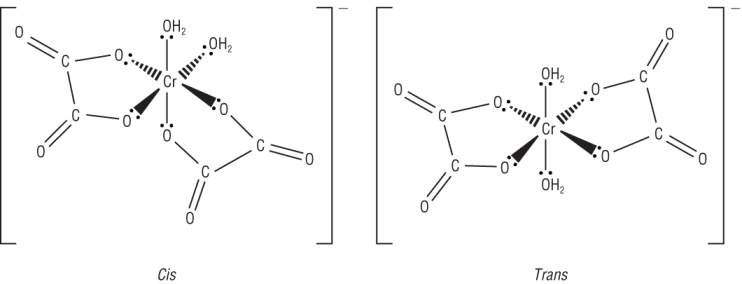CIS = 2 monodentate ligands are at 90o to each other TRANS = 2 monodentate ligands are at 180o to each other

Hexadentate ligand:

• Hexa = 6
• Hexadentate ligands is a molecule with 6 lone pairs of electrons forming 6 coordinate bonds: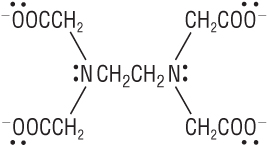It is used to bind to metal ions, which reduces the concentration of those metal ions in solution. Used in detergents to soften water - binds to Ca and Mg ions. Binds to metals in foods stopping those metals catalyse oxidation Added to blood samples stopping it clotting Used to treat mercury poisoning. These processes are called chelating EDTA4-

Optical isomers:

• These occur in complex ions too: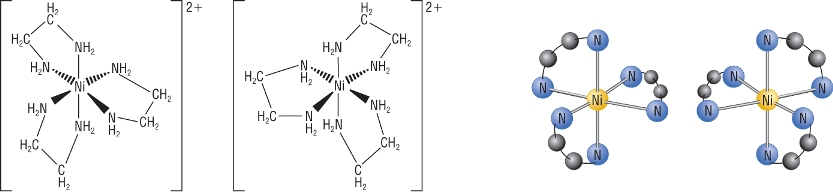• The requirements are:

1)  3 bidentate ligands

2)  2 bidentate ligands / 2 monodentate ligands in the cis isomer only

3)  Hexadentate ligand

• These combinations will give you 2 non - superimposable images of each other.
• They will rotate plane polarised light.

Qu 1  P213

Ligand substitution in complex ions

Ligand substitution reactionsThese are reactions when one ligand in a complex ion is substituted with another

1)  The reaction of aq copper (II) ions and ammonia

 [Cu(H2O)6]2+(aq) + 4NH3(aq) D [Cu(NH3)4(H2O)2]2+(aq) + 4H2O(l) Pale Blue sol Deep Blue sol
 4 of the H2O ligands are replaced with 4 NH3 ligands 6 ligands arranged in a distorted octahedral arrangement due to the longer Cu - N bondsIn practise: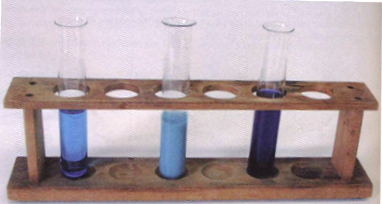When you start adding ammonia you get the distinguishable pale blue precipitate of Cu2+ ions with hydroxide ions first. On further addition of ammonia the deep blue colour appears. This is because aq ammonia contains hydroxide ions and these react first:

Ammonia contains:

 H2O(l) + NH3(aq) D NH4+(aq) + OH-(aq)

a)  Copper ions react with hydroxide ions:

 Cu2+(aq) + 2OH-(aq) à Cu(OH)2(s) Pale Blue Pale Blue ppt

b)  Copper ions react with ammonia:

 [Cu(H2O)6]2+(aq) + 4NH3(aq) D [Cu(NH3)4(H2O)2]2+(aq) + 4H2O(l) Pale Blue sol Deep Blue sol

Summary:[Cu(H2O)6]2+ Cu(OH)2 [Cu(NH3)4(H2O)2]2+

2)  The reaction of aq copper (II) ions and hydrochloric acid

 [Cu(H2O)6]2+(aq) + 4Cl-(aq) D [CuCl4]2-(aq) + 6H2O(l) Pale Blue sol Yellow sol
 6 H2O ligands are replaced with 4 Cl- ligands. This is because the Cl- ligand is larger than the H2O ligand. The Cl- ligand also offers more repulsion. The [CuCl4]2- complex will have a tetrahedral shape around the central ion. The equilibrium can be reversed by the addition of water. The green intermediate is due to the mixing of the colours blue and yellow colours.

3)  The reaction of cobalt (II) ions and conc hydrochloric acid

 [Co(H2O)6]2+(aq) + 4Cl-(aq) D [CoCl4]2-(aq) + 6H2O(l) Pink sol Dark blue sol
 6 H2O ligands are replaced with 4 Cl- ligands. Concentrated HCl is used to provide a high concentration of Cl- ions / ligands The equilibrium can be reversed by the addition of water.

Qu 1-3 P215

Ligand substitution and stability constants

Haemoglobin and ligand substitution

Haemoglobin - is made from 2 parts

a)  Haem:                                                                                            b)  Globin: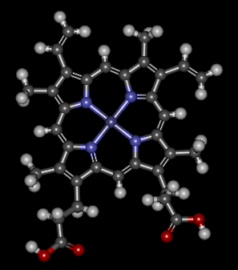Haem is a non protein molecule complex that can bind to Fe2+ ions. It forms 4 dative covalent bonds to Fe2+. Its coordination number is 4 and binds in a square planar arrangement. This allows 2 further dative covalent bonds - 1 above and 1 below.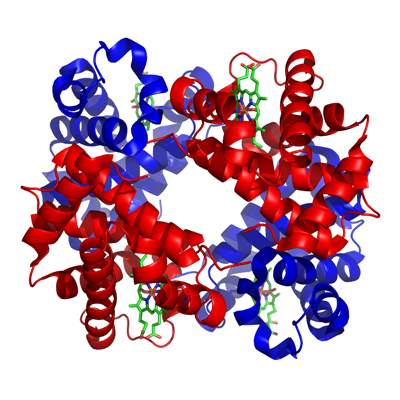Globin is made from 4 proteins joined together in a polypeptide. They are optical isomers of each other. Each protein forms a dative covalent bond to the Fe2+ This leaves 1 area free for a dative covalent bond.

A simpler picture: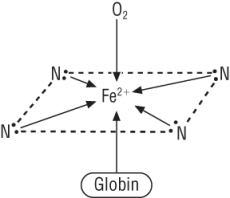Looking at one of the haem complexes which forms a further dative covalent bond with one of the proteins in globin. A space is left for a further dative covalent bond.  This is where oxygen forms a dative covalent bond in order to be transported around the body. It also forms a complex with carbon dioxide which is transported back to the lungs.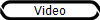Carbon monoxide - the silent killer

• Carbon monoxide can bind to haemoglobin sites in exactly the same way as oxygen.
• Carbon monoxide however binds more favourable than oxygen.
• This results in the tissues being starved of oxygen.
• To make matters worse - once carbon monoxide binds to the haem site, it cannot be removed.
• That haem site is now useless.
• The complex ion formed is much more stable with carbon monoxide than with oxygen.
• This reaction is a simple ligand substitution reaction.

Stability constants

• In equilibria, Kc constants were used to show the proportions of products : reactants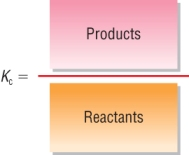Kc = [PRODUCTS]p [REACTANTS]r
• This principle can be used to gauge which ligand is most likely to bind with a transition metal forming the most stable complex ion:

Example:

 [Co(H2O)6]2+(aq) + 4Cl-(aq) D [CoCl4]2-(aq) + 6H2O(l)
• It is possible to write an equilibrium expression, Kc:
 Kc = [ [CoCl4]2- ]     [ [H2O] ]6 [ [Co(H2O)6]2+ ]   [ [Cl-] ]4
• However, the concentration of water is removed as it is dissolved in water.
• This means the concentration of water is very large and therefore virtually constant:
 Kc = [ [CoCl4]2- ] [ [Co(H2O)6]2+ ]   [ [Cl-] ]4
• Remember if Kc > 1  products predominate
• Remember if Kc < 1  reactants predominate
• It gives you a guide as to which ligand is the most stable
• Because of this we re-name the Equilibrium constant to Stability constant:
 Kstab = [ [CoCl4]2- ] [ [Co(H2O)6]2+ ]   [ [Cl-] ]4
• The units are worked out in the same way as in the equilibria unit
• mol-4  dm+12

The value of Kstab:

• These stability constants are all arbitrarily compared with water.
• The tables give stability constants indicating the stability of that complex with that ion compare with that ion's complex with water.
• The larger the stability constant = the more stable it is (ie it is the one that will form)

Example:

 [Cu(H2O)6]2+(aq) + 4Cl-(aq) D [CuCl4]2-(aq) + 6H2O(l)
• At 25oC the following equilibrium mixture contained:

[ [Cu(H2O)6]2+ ]  =  1.17  x  10-5  mol dm-3  and  [ Cl-]  =  0.08  mol dm-3   and Kstab = 4.17  x  10-5  mol-4 dm12

• Write an expression for Kstab and calculate the concentration of [CuCl4]2- :
 Kstab = [ [CuCl4]2- ] [ [Cu(H2O)6]2+ ]   [ [Cl-] ]4
• Put in values:
 4.17  x  10-5 = [ [CuCl4]2- ] 1.17  x  10-5  x  0.08
• Rearrange and calculate:

[ [CuCl4]2- ] =  2.00 mol dm-3

Qu 1 - 3  P217

Redox titrations

Oxidation and reduction in transition metal chemistry

• Remember transition metals have variable oxidation states.
• This means that their ions are able to undergo oxidation and reduction

MnO4-  and Fe2+

• Iron can exist as Fe2+ and Fe3+ with the most stable being Fe3+:
 Fe2+(aq) à Fe3+(aq) + e- 1
• Manganese can exist as many ions but the most common are MnO4- (+7) and Mn2+
• In acidic conditions MnO4- is a strong oxidising agent.
• This means that MnO4- is itself reduced to Mn2+ ions:
 MnO4-(aq) + 8H+(aq) + 5e- à Mn2+(aq) + 4H2O(l) 2
• If you look at the 2 half reactions:  Reaction 1 has to occur 5 x for every one of reaction 2.
• When you put the 2 together:
 MnO4-(aq) + 8H+(aq) + 5Fe2+(aq) à Mn2+(aq) + 4H2O(l) + 5Fe3+(aq)
 Purple Colourless
• Due to the colour changes associated with this redox reaction it is possible to calculate the % composition of a metal in a solid sample of compound or alloy:

Carrying out redox titrations

• These are carried out in exactly the same way as an acid - base titration.
• The only difference is that instead of neutralisation, an oxidising agent is titrated against a reducing agent.
• The great thing about using transition metals is that they are often associated with a colour change, (just as indicators are used in acid base titrations).
 MnO4-(aq) + 8H+(aq) + 5Fe2+(aq) à Mn2+(aq) + 4H2O(l) + 5Fe3+(aq)
 Purple Colourless
• In this titration, the 'end point' would be the first permanent pink colour (indicating that all Fe2+ ions had been oxidised).
• The MnO4- ions are used in a very low concentration as Mn2+ ions are a very pale pink and in very low concentrations this colour cannot be seen.
• This is then followed by a moles calculation:

Worked example:

25.0cm3 of a solution of iron (II) salt required 23.00cm3 of 0.0200 mol dm-3 potassium manganate (VII) for complete oxidation in acidic solution.

 MnO4-(aq) + 8H+(aq) + 5Fe2+(aq) à Mn2+(aq) + 4H2O(l) + 5Fe3+(aq) 23cm3 1 : 5 25cm3 0.02 mol dm-3 Conc = Mol x 1000/v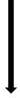= 2.3 x 10-3 x 1000/25 = 0.092 mol dm-3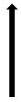Moles = C x V/1000 = 0.02 x 23/1000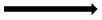= 4.6 x 10-4 x 5 = 2.3 x 10-3

Qu 1 - 2  P 219

Examples of redox titrations

• The common uses of these redox titrations are:

1)  Calculate the Mr and formula of an iron (II) salt

2)  Calculating the % mass of iron in iron tablets

3)  Calculating the purity of iron samples

4)  Applying your knowledge to unfamiliar redox reactions / titrations

• Some examples:

1)  Calculate the Mr and formula of an iron (II) salt

2.950g of hydrated iron (II) sulphate, FeSO4.xH2O, was dissolved in 50cm3 of sulphuric acid.  This was made up to 250cm3 with distilled water.

25cm3 of this was titrated with 0.01 mol dm-3 KMnO4 and 21.20cm3of this was used

 MnO4-(aq) + 8H+(aq) + 5Fe2+(aq) à Mn2+(aq) + 4H2O(l) + 5Fe3+(aq) 21.20cm3 25cm3 0.01 mol dm-3 (misprint in book)Moles = C x V/1000 1 : 5 = 0.01 x 21.20/1000= 2.12 x 10-4 x 5 = 1.06 x 10-3
• Number of moles of Fe2+ = 1.06 x 10-3 in 25cm3 of solution ( x 10 in 250cm3)
• Number of moles of Fe2+ = 0.0106 x 10-3 in 250cm3 of solution
• Mr  =  mass / moles    2.950 / 0.0106  =  278.3 gmol-1
• For Mr of all waters, deduct the Mr of FeSO4  =  278.3  -  151.9  =  126.4
• No waters  =  126.4  /  18   =   7
• Formula:  FeSO4.7H2O

2)  Calculating the % mass of iron in iron tablets

A multivitamin tablet has a mass of 0.325g and contains iron.  The powdered tablet was dissolved in some water and sulphuric acid.

12.10cm3 of 0.002 mol dm-3 KMnO4 was titrated until the first permanent pink colour.

 MnO4-(aq) + 8H+(aq) + 5Fe2+(aq) à Mn2+(aq) + 4H2O(l) + 5Fe3+(aq) 12.10cm3 Mass = moles x Ar 0.002 mol dm-3 = 1.21 x 10-4 x 55.8= 0.00675gMoles = C x V/1000 1 : 5 = 0.002 x 12.10/1000= 2.42 x 10-5 x 5 = 1.21 x 10-4
• % Fe by mass = (Mass of element  /  Mass of tablet)  x  100
• % Fe by mass = (0.00675  /  0.325)  x  100
• % Fe = 2.08%4)  Applying your knowledge to unfamiliar redox reactions / titrations

25cm3 portion of H2O2 was made up to 250cm3 with distilled water.

25cm3 portions of this was acidified and titrated with 0.0200 mol dm-3 KMnO4, 38.00cm3 of this was required to completely oxidise the hydrogen peroxide.

Calculate the original concentration of the hydrogen peroxide.  The following equation represents the oxidation of hydrogen peroxide

 H2O2(aq) à O2(g) + 2H+ + 2e- 1
• Remember the manganate reaction:
 MnO4-(aq) + 8H+(aq) + 5e- à Mn2+(aq) + 4H2O(l) 2
• If you look at the 2 half reactions:  Reaction 1 has to occur 5 x for every 2 x of reaction 2.
• When you put the 2 together:
 2MnO4-(aq) + 16H+(aq) + 5H2O2(aq) à 2Mn2+(aq) + 8H2O(l) + 5O2(aq) + 10H+(aq)
• Note that some of the H+ ions will cancel out:
 2MnO4-(aq) + 6H+(aq) + 5H2O2(aq) à 2Mn2+(aq) + 8H2O(l) + 5O2(aq)
 Purple Colourless
• Now tackle the questions:

25cm3 portion of H2O2 was made up to 250cm3 with distilled water.

25cm3 portions of this was acidified and titrated with 0.0200 mol dm-3 KMnO4, 38.00cm3 of this was required to completely oxidise the hydrogen peroxide.

Calculate the original concentration of the hydrogen peroxide.  The following equation represents the oxidation of hydrogen peroxide

 2MnO4-(aq) + 6H+(aq) + 5H2O2(aq) à 2Mn2+(aq) + 8H2O(l) + 5O2(aq)
 38.00cm3 25cm3 0.02 mol dm-3 C = moles  x  1000 / V=  1.9 x 10-3 x 1000 / 25 = 0.0760 mol dm-3Moles = C x V/1000 2 : 5 = 0.02 x 38.00/1000= 7.6 x 10-4 x 2.5 = 1.9 x 10-3
• Remember this was diluted by a factor of 10 at the beginning
• Concentration of original = 0.760 mol dm-3

Qu 1 - 2  P221

Redox titrations - iodine and thiosulphate

• The reaction between iodine, I2 and thiosulohate, S2O32- is a common redox reaction used in analysis.
• It can be used in any reaction where iodine, I is produced or where iodine, I2 can be liberated from iodide, I-.
• The redox reaction is:
 2S2O32-(aq) + I2(aq) à 2I-(aq) + S4O62-(aq) BROWN COLOURLESS
• These analysis reaction is usually used where iodine, I2 is liberated from its iodide, I-.
• This makes it a more difficult moles calculation as you will be using 2 reactions.

1)  Iodine is liberated from it ions - using an oxidising agent (redox reaction):

2I-       à         I2    +    2e-

a)  Copper (II):  Cu2+

b)  Dichromate:  Cr2O72-

c)  Chlorate:  ClO-

2)  The liberated iodine is titrated with a known concentration of thiosulphate,

 2S2O32-(aq) + I2(aq) à 2I-(aq) + S4O62-(aq) BROWN COLOURLESS
• Starch solution is usually added near the end point to enhance the iodine colour - blue black colour.
• From the titration results you are able to calculate amount of iodine and hence the amount of the oxidising agent.

Example:

30cm3 of bleach was added to an excess of iodide ions, I- in sulphuric acid.

Bleach contains chlorate (I) ions, ClO-.

In the presence of acid, chlorate (I) ions oxidise iodide ions, I- to iodine, I2

The iodine formed was titrated against 0.200 Mol dm-3 of sodium thiosulphate, 29.45cm3 was required.

Calculate the concentration of chlorate ions, ClO- in the bleach:

 ClO-(aq) + 2H+(aq) + 2I-(aq) à Cl-(aq) + I2(laq) + H2O(aq)  30.00cm3 BROWN

Then:

 2S2O32-(aq) + I2(aq) à 2I-(aq) + S4O62-(aq) BROWN COLOURLESS 0.200 Mol dm-3 29.45 cm3Moles = C x V/1000 2 : 1 = 0.200 x 29.45/1000= 5.890 x 10-3 x 0.5 = 2.945 x 10-3
• The 2.945 x 10-3 moles of iodine was liberated in reaction 
• This means this is the moles of ClO- = 2.945  x 10-3
• So now a concentration can be calculated:  C = moles  x  1000 / vol,    = 2.945 x 10-3   x  1000 / 30,   =  0.0982 Mol dm-3

Estimating the copper content of solutions and alloys:

1)  Iodine is liberated from it ions - using Cu2+ ions:

• If a solution contains copper (II) ions, Cu2+ and is mixed with iodide ions, I- the following reaction occurs:
 2Cu2+(aq) + 4I-  2(aq) à 2CuI(s) + I2(aq) COLOURLESS WHITE SOLID BROWN SOLn
• The white ppt appears light brown due to the colour of the iodine in solution.

2)  The liberated iodine is titrated with a known concentration of thiosulphate,

 2S2O32-(aq) + I2(aq) à 2I-(aq) + S4O62-(aq) BROWN COLOURLESS
• Starch solution is usually added near the end point to enhance the iodine colour - blue black colour
• From the titration results you are able to calculate amount of iodine and hence the amount of the Cu2+ ions.

Copper metal:

• To estimate the amount of copper metal you must first convert that to copper ions before this whole process begins.
• This is done using concentrated Nitric acid, HNO3
• This adds a 3rd step to the reaction:

1)  Convert Cu to Cu2+:

 conc. HNO3 Cu(s) à Cu2+(aq) + 2e- 

2)  Liberate iodine:

 2Cu2+(aq) + 4I-  2(aq) à 2CuI(s) + I2(aq) COLOURLESS WHITE SOLID BROWN SOLn

3)  Titrate with a known concentration of thiosulphate,

 2S2O32-(aq) + I2(aq) à 2I-(aq) + S4O62-(aq) BROWN COLOURLESS
• You then have to work your mole calculation backwards through the reactions as before.

Ratio:                  S2O32-  :  I2  :  Cu2+  :  Cu

2        :  1   :    2       :   2

Overall ratio is      1                 :             1

Example:

0.500g of bronze was reacted with nitric acid giving a Cu2+ solution.

The solution was reacted with iodide ions, I- forming a solution of iodine, I2.

This iodine solution, I2 was then titrated with 0.200 mol dm-3 solution of sodium thiosulphate, S2O32-.  22.40cm3 of this was required.

Calculate the % copper in brass:

1)  Convert Cu in brass to Cu2+:

 conc. HNO3 Cu(s) à Cu2+(aq) + 2e- 

2)  Liberate iodine:

 2Cu2+(aq) + 4I-  2(aq) à 2CuI(s) + I2(aq) COLOURLESS WHITE SOLID BROWN SOLn

3)  Titrate with a known concentration of thiosulphate,

 2S2O32-(aq) + I2(aq) à 2I-(aq) + S4O62-(aq) BROWN COLOURLESS 0.200 Mol dm-3 22.4 cm3Moles = C x V/1000 = 0.200 x 22.40/1000 = 4.48 x 10-3
• Overall the ratio is 1  :  1
• This means that the moles of Cu = 4.48  x 10-3
• Mass of copper  =  Moles  x  Ar,      4.48  x  10-3  x  63.5  =  0.28448g
• % mass Cu in brass  =  (0.28448  /  0.500)  x  100  =  56.9%

Qu 1 - 2  P 223 / Qu 9  P 225 / Qu's 226 - 229